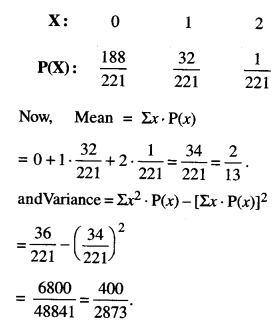Here we are providing Class 12 Maths Important Extra Questions and Answers Chapter 13 Probability. Class 12 Maths Important Questions are the best resource for students which helps in Class 12 board exams.

## Class 12 Maths Chapter 13 Important Extra Questions Probability

### Probability Important Extra Questions Very Short Answer Type

Question 1.
If A and B are two independent event, prove that A’ and B are also independent.
(C.B.S.E. Sample Paper 2018-19)
Solution:
Since A and B are independent events, [Given]
.-. P (A ∩ B) = P (A) . P(B) …(1)
Now P(A’∩B) = P (B) – P (A ∩ B)
= P(B) – P (A) P( ∩ B) [Using (1)]
= (1 – P(A)) P(B) = P(A’) P(B).
Hence, A’ and B are independent events.

Question 1.
One card is drawn from a pack of 52 cards so that each card is equally likely to be se-lected. Prove that the following cases are in-dependent :
(a) A : “The card drawn is a spade”
B : “The card drawn is an ace.”
(N.C.E.R.T.)
(b) A : “The card drawn is black”
B : “The card drawn is a king.”
(.N.C.E.R.T.)
Solution:
(a) P(A) =$$\frac{13}{52}=\frac{1}{4}$$,P(B) = $$\frac{4}{52}=\frac{1}{13}$$
P(A∩B) = $$\frac{1}{52}=\frac{1}{4} \cdot \frac{1}{13}$$ = p(A).p(B)
Hence, the events A and B are independent

(b) P(A) = $$\frac{26}{52}=\frac{1}{2}$$, P(B) = $$\frac{4}{52}=\frac{1}{13}$$
P(A∩B) = $$\frac{2}{52}=\frac{1}{26}=\frac{1}{2} \cdot \frac{1}{13}$$ = P(A).P(B)
Hence, the events A and B are independent

Question 3.
A pair of coins is tossed once. Find the probability of showing at least one head.
Solution:
S, Sample space = {HH, HT, TH, TT}
where H ≡ Head and T ≡ Tail.
∴ P (at least one head) = $$\frac { 3 }{ 4 }$$ .

Question 4.
P(A) = 0.6, P(B) = 0. 5 and P(A/B) = 0.3, then find P(A∪ B)
(C.B.S.E. Sample Paper 2018-19)
Solution:
We have: P(A/B) = 0.3
$$\frac{P(A \cap B)}{P(B)}$$ = 0.3
$$\frac{P(A \cap B)}{0.5}$$ = 0.3
P (A ∩ B) = 0.5 x 0.3 = 0.15.
Now, P(A∪B) = P(A) + P(B) – P(A ∩ B)
= 0.6 + 0.5 – 0. 15
Hence, P (A ∪ B) = 1.1 – 0.15 = 0.95.

Question 5.
One bag contains 3 red and 5 black balls. Another bag contains 6 red and 4 black balls. A ball is transferred from first bag to the second bag and then a ball is drawn from the second bag. Find the probability that the ball drawn is red. (C.B.S.E. Sample Paper 2018-19)
Solution:
P(Red transferred and red drawn or black trans¬ferred red drawn)
$$\begin{array}{l} =\frac{3}{8} \times \frac{7}{11}+\frac{5}{8} \times \frac{6}{11} \\ =\frac{21}{88}+\frac{30}{88}=\frac{51}{88} \end{array}$$

Question 6.
Evaluate P(A ∪ B), if 2P(A) = P(B) = $$\frac { 5 }{ 13 }$$ and P(A/B) = $$\frac { 2 }{ 5 }$$ (C.B.S.E. 2018 C)
Solution:
P(A/B) = $$\frac{\mathrm{P}(\mathrm{A} \cap \mathrm{B})}{\mathrm{P}(\mathrm{B})}$$ P(A∩B) = $$\frac{2}{11}$$
P(A∪B) = P(A) + P(B) – (A ∩ B)
= $$\frac{5}{26}+\frac{5}{13}-\frac{2}{13}=\frac{11}{26}$$

### Probability Important Extra Questions Short Answer Type

Question 1.
Given that A and B are two independent events such that P(A) = 0.3 and P(B) = 0.5. Find P(A/B). (C.B.S.E. 2019 C)
Solution:
We have:
P(A)= 0.3 and P(B) = 0.5.
Now P(A ∩ B) = P(A). P(B)
[∵A and B are independent events]
= (0.3) (0.5) = 0.15.
Hence, P(A/B) = $$\frac{\mathrm{P}(\mathrm{A} \cap \mathrm{B})}{\mathrm{P}(\mathrm{B})}=\frac{0.15}{0.5}$$ =0.3.

Question 2.
A bag contains 3 white and 2 red balls, another bag contains 4 white and 3 red balls. One ball is drawn at random from each bag.
Find the probability that the balls drawn are one white and one red.
(C.B.S.E. 2019 C)
Solution:
Reqd. probability
= P(White, Red) + P (Red, White)
$$\frac{3}{5} \times \frac{3}{7}+\frac{2}{5} \times \frac{4}{7}=\frac{9}{35}+\frac{8}{35}=\frac{17}{35}$$

Question 3.
The probabilities of A, B and C solving a problem independently are $$\frac{1}{2}, \frac{1}{3}$$ and $$\frac{1}{4}$$ respectively. If all the three try to solve the problem independently, find the probability that the problem is solved. (C.B.S.E. 2019 C)
Solution:
Given: P(A) = $$\frac{1}{2}$$, P(B) = $$\frac{1}{3}$$ and P(C) = $$\frac{1}{4}$$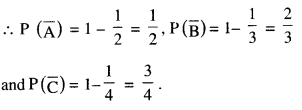Probability that the problem is solved
= Probability that the problem is solved by at least one person
= 1 – $$\mathrm{P}(\overline{\mathrm{A}}) \mathrm{P}(\overline{\mathrm{B}}) \mathrm{P}(\overline{\mathrm{C}})$$
= 1 – $$\frac{1}{2} \times \frac{2}{3} \times \frac{3}{4}$$= 1 – $$\frac{1}{4}=\frac{3}{4}$$

Question 4.
A die marked 1, 2, 3 in red and 4, 5, 6 in green is tossed. Let A be the event “number is even” and B be the event “number is marked red”. Find whether the events A and B are independent or not. {Delhi 2019)
Solution:
Here, A : number is even i.e.,
A = {2,4,6}
and B : number is red i.e.,
B = {1,2,3}
∴ P(A) = $$\frac{3}{6}=\frac{1}{2}$$ and P(B) = $$\frac{3}{6}=\frac{1}{2}$$
And,
P(A ∩ B) = P(Number is even and red) = $$\frac { 1 }{ 6 }$$ .
Thus, P(A ∩ B) ≠ P(A). P(B)
[∵ $$\frac{1}{6} \neq \frac{1}{2} \times \frac{1}{2}$$ ]
Hence, the events A and B are not indepedent.

Question 5.
A die is thrown 6 times. If “getting an odd number” is a success, what is the probability of (i) 5 successes (ii) at most 5 successes? (Delhi 2019)
Solution:
Probability of getting an odd number is one 3 1
trial = $$\frac{3}{6}=\frac{1}{2}$$ = p ( say)
Probability of getting an even number in one 3
trial = $$\frac{3}{6}=\frac{1}{2}$$ = g(say) o l
Also, n = 6.

(i) P(5 successes) = P(5) = 6C5 q1 p5
(ii) P(at most 5 successes)
= P(0) + P(1) + … + P(5) = 1 – P(6)
= 1 – 6C6 q0 p6
= 1 – $$\frac{1}{64}=\frac{63}{64}$$

Question 6.
The random variable ‘X’ has a probability distribution P(X) of the following form, where ‘k’ is some number :
$$\mathbf{P}(\mathbf{X}=\boldsymbol{x})=\left\{\begin{array}{l} \boldsymbol{k}, \text { if } \boldsymbol{x}=\mathbf{0} \\ 2 k, \text { if } x=1 \\ 3 k, \text { if } x=2 \\ 0, \text { otherwise. } \end{array}\right.$$
Determine the value of ‘P. (Outside Delhi 2019)
Solution:
We have : P(X = 0) + P(X = 1) + P(X = 2) = 1
⇒ k + 2k + 3k = 1
⇒ 6k = 1.
Hence, k = $$\frac { 1 }{ 6 }$$.

Question 7.
Out of 8 outstanding students of a school, in which there are 3 boys and 5 girls, a team of 4 students is to be selected for a quiz competiton. Find the probability that 2 boj and 2 girls are selected. (Outside Delhi 2019)
Solution:
Read, probability = $$\frac{{ }^{3} \mathrm{C}_{2} \times{ }^{5} \mathrm{C}_{2}}{{ }^{8} \mathrm{C}_{4}}$$
= $$\frac{3 \times 10}{70}=\frac{3}{7}$$

Question 8.
12 cards numbered 1 to 12 (one number on one card), are placed in a box and mixed up thoroughly. Then a card is drawn at ran¬dom from the box. If it is known that the number on the drawn card is greater than 5, find the probability that the card bears an odd number. {Outside Delhi 2019)
Solution:
Let the events be as :
A : Card bears an odd number.
B : Number on the card is greater than 5.
A∩B = {7, 9, 11}.
Hence, P(A/B) = $$\frac{P(A \cap B)}{P(B)}$$
= $$\frac{3 / 12}{7 / 12}=\frac{3}{7}$$

Question 9.
The probability of solving a specific problem independently by A and B are $$\frac { 1 }{ 3 }$$ and $$\frac { 1 }{ 5 }$$ respectively. If both try to solve the problem independently, find the probability that the problem is solved.
(iOutside Delhi 2019)
Solution:
P (Problem is solved)
= 1 – P (Problem is not solved)
= 1 – $$\left(1-\frac{1}{3}\right)\left(1-\frac{1}{5}\right)$$
= 1 – $$\frac{2}{3} \times \frac{4}{5}$$
= 1 – $$\frac{8}{15}=\frac{7}{15}$$

### Probability Important Extra Questions Long Answer Type 1

Question 1.
A black and a red die are rolled together. Find the conditional probability of obtaining the sum 8, given that the red die resulted in a number less than 4. (C.B.S.E. 2018)
Solution:
Let the events be as:
E : Sum of numbers is 8
F : Number of red die less than 4.
E : {(2, 6), (3, 5), (4, 4), (5, 3), (6, 2)}
F = {(1, 1), (2, 1), … (6, 1), (1, 2), (2, 2), … (6, 2), (1, 3), (2, 3), … (6, 2) (6, 3)}
and E ∩ F = {(5, 3), (6, 2)}
P(E) = $$\frac{5}{36}$$, P(F) = $$\frac{18}{36}$$
and P(E ∩ F) = $$\frac{2}{36}$$.
Hence, P(E/F) = $$\frac{\mathrm{P}(\mathrm{E} \cap \mathrm{F})}{\mathrm{P}(\mathrm{F})}=\frac{2 / 36}{18 / 36}$$
= $$\frac{2}{18}=\frac{1}{9}$$

Question 2.
Two numbers are selected at random (with-out replacement) from the first five positive integers. Let X denote the larger of the two numbers obtained. Find the mean and vari-ance of X.
Solution:
The first five positive integers are 1, 2, 3, 4 and 5.
We select two positive numbers in 5 x 4 = 20 way.
Out of three, two numbers are selected at ran-dom.
Let ‘X’ denote the larger of the two numbers.
X can be 2, 3, 4 or 5.
∴ P (X = 2) = P (Larger number is 2)
{(1, 2), (2,1)} = $$\frac{2}{20}$$
Similarly, P(X = 3) = $$\frac{4}{20}$$ ,
P(X = 4) = $$\frac{6}{20}$$
and P(X = 5) = $$\frac{8}{20}$$
Hence, the probability distribution is: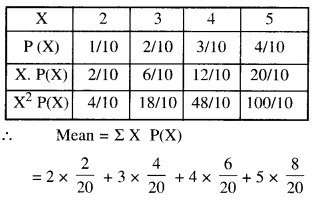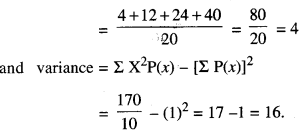Question 3.
The probabilities of two students A and B coming to the school in time are $$\frac{3}{7}$$ and $$\frac{5}{7}$$ respectively. Assuming that the events, ‘A coming in time’ and ‘B coming in time’ are independent, find the probability of only one of them coming to the school in time. (A.I.C.B.S.E. 2013)
Solution:
We have : P(A) = Probability of student A coming to school in time = $$\frac{3}{7}$$
P(B) = Probability of student B coming to school in time = $$\frac{5}{7}$$
∴ $$\mathrm{P}(\overline{\mathrm{A}})=1-\frac{3}{7}=\frac{4}{7}$$
and $$\mathrm{P}(\overline{\mathrm{B}})=1-\frac{5}{7}=\frac{2}{7}$$
∴ Probability that only one of the students coming to school in time
= P(A ∩ $$\overline{\mathrm{B}}$$) + P( $$\overline{\mathbf{A}}$$ ∩B)
= P(A)P($$\overline{\mathrm{B}}$$) + P($$\overline{\mathrm{B}}$$)PB)
[∵ A and B are independent => A and $$\overline{\mathrm{B}}$$ and $$\overline{\mathrm{A}}$$ and B are also independent]
= $$\left(\frac{3}{7}\right)\left(\frac{2}{7}\right)+\left(\frac{4}{7}\right)\left(\frac{5}{7}\right)=\frac{26}{49}$$

Question 4.
A speaks truth in 80% cases and B speaks truth in 90% cases. In what percentage of cases are they likely to agree with each other in stating the same fact? (C.B.S.E. Sample Paper 2019-20)
Solution:
P(A) = $$\frac{80}{100}=\frac{4}{5}$$
and P( B) = $$\frac{90}{100}=\frac{9}{10}$$
P($$\overline{\mathrm{A}}$$) = 1 – P(A) = 1 – $$\frac{4}{5}=\frac{1}{5}$$
P($$\overline{\mathrm{B}}$$) = 1 – P(B) = 1 – $$\frac{9}{10}=\frac{1}{10}$$
∴ P(Agree) = P(Both speak the truth or both tell a lie)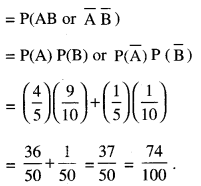Hence, the reqd. percentage = 74%.

Question 5.
A problem in Mathematics is given to three students whose chances of solving it are $$\frac{1}{2}, \frac{1}{3}, \frac{1}{4}$$. What is the probability in the
following cases?
(i) that the problem is solved
(ii) only one of them solves it correctly
(iii) at least one of them may solve it.
Solution:
Let A, B, C be three events when a problem in
Mathematics is solved by three students.
Given : P(A) = $$\frac{1}{2}$$, P(B) = $$\frac{1}{3}$$, P(C) = $$\frac{1}{4}$$.
.-. P(A) = 1 – $$\frac{1}{2}$$ = $$\frac{1}{2}$$, P(B) = 1 – $$\frac{1}{3}$$ = $$\frac{2}{3}$$
and P(C) = 1 – $$\frac{1}{4}$$ = $$\frac{3}{4}$$.

(i) Probability that the problem is solved = Probability that the problem is solved by at least one student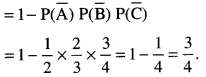(ii) Probability that only one solves it correctly = P(Aninc)+P(AnBnc)+P(AnBnc)(iii) Probability that atleast one of them may solve the problem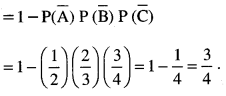Question 6.
In a set of 10 coins, 2 coins with heads on both sides. A coin is selected at random from this set and tossed five times. Of all the five times, the result was head, find the probability that the selected coin had heads on both sides. (A.I. C.B.S.E. 2015)
Solution:
Let the events be :
E1 : Selecting a coin with two heads
E2 : Selecting a normal coin and A : The coin falls head all the times.
Since E1 and E2 are mutually exclusive and by the data given in the problem, we have :
P(E1) = $$\frac{2}{10}=\frac{1}{5}$$
P(A/E2) = $$\frac{8}{10}=\frac{4}{5}$$ = P(A/E1) = 1
P(A/E1) = 1
P(A/E2) = $$\frac{1}{2} \times \frac{1}{2} \times \frac{1}{2} \times \frac{1}{2} \times \frac{1}{2}=\frac{1}{32}$$
Now P(A) = P(A ∩ E1) + P(A ∩ E2)
= P(E1) P(A/ E1) + P(E2) P(A/ E2)
= $$\frac{1}{5}+\frac{1}{40}=\frac{8+1}{40}=\frac{9}{40}$$

Question 7.
A person playsa game of tossing a coin thrice. For each head he is given XI by the organiser of the game and for each tail he has to give ₹1.50 to the organiser. Let ‘X’ denote the amount gained or lost by the person. Show that ‘X’ is a random variable and exhibit it as a function on the sample space of the experiment. (N.C.E.R.T.)
Solution:
Since ‘X’ is a number whose values are defined by the outcomes of the random experiment,
∴ ‘X’ is a random variable.
Now sample space is given by :
S = {HHH, HHT, HTH, THH, HIT, THT, TTH, ITT},
where H = Head and T = Tail.
Thus X (HHH) = 2 x 3 = ₹6
X (HHT) = X (HTH) = X (THH)
= 2×2-lxl.5 = ₹ 2.50
X (HTT) = X (THT) = X (TTH)
= lx2-2xl.5 = -₹l
and X (TTT) = -(3xl.5) = – ₹4.50.
Thus for each element of S, X takes a unique value.
∴ ‘X’ is a function on the sample space S having range = {6, 2.50, – 1, – 4.50}.

Question 8.
Two numbers are selected at random (without replacement) from first 7 natural numbers. If X denotes the smaller of the two numbers obtained, find the probability distribution of X. Also, find mean of the distribution.
(C.B.S.E. Sample Paper 2019-20)
Solution:
Let ‘X’ be the smaller of the two numbers obtained.
Thus, X takes values 1, 2, 3, 4, 5 and 6.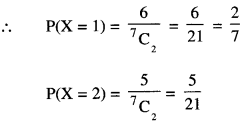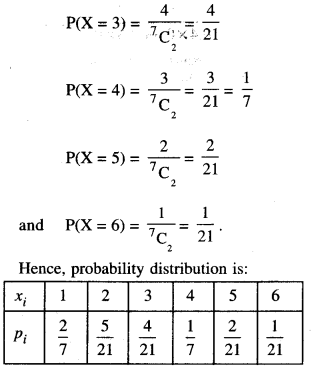(ii) We have :Question 9.
Two cards are drawn simultaneously (with-out replacement) from a well shuffled pack of 52 cards. Find the mean and variance of the number of red cards. (A.I.C.B.S.E. 2012)
Solution:
Here ‘X’ takes values 0, 1, 2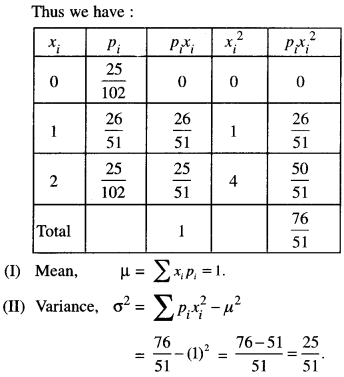Question 10.
There is a group of 50 people who are patriotic out of which 20 believe in non-violence. Two persons are selected at random out of them, write the probability distribution for the selected persons who are non-violent. Also find the mean of the distribution.
Solution:
Let ‘X’ be the number of non-violent persons.
Here ‘X’ takes values 0, 1,2.
Thus no. of non-violent persons = 20
and no. of violent persons = 50 – 20 = 30.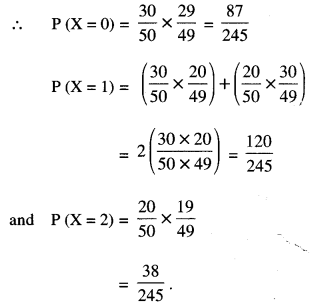Hence, probability distribution is given by :Question 11.
Two groups are competing for the positions of the Board of Directors of a corporation. The probabilities that the first and second groups will win are 0.6 and 0.4 respectively. Further, if the first group wins, the probability of introducing a new product is 0.7 and the corresponding probability is 0.3 if the second group wins. Find the probability that the new product introduced was by the second group. (C.B.S.E. 2018 C)
Solution:
Let E1 = First group wins, E2 = Second group wins
H = Introduction of new product.
P(E1) = 0.6, P(E2) = 0.4
P(H/E2) = 0.3 P(H/E1) = 0.7
Now,
P(E2/H) = $$\frac{\mathrm{P}\left(\mathrm{E}_{2}\right) \mathrm{P}\left(\mathrm{H} / \mathrm{E}_{2}\right)}{\mathrm{P}\left(\mathrm{E}_{2}\right) \mathrm{P}\left(\mathrm{H} / \mathrm{E}_{2}\right)+\mathrm{P}\left(\mathrm{E}_{1}\right) \mathrm{P}\left(\mathrm{H} / \mathrm{E}_{1}\right)}$$

Question 12.
From a lot of 20 bulbs which include 5 defectives, a sample of 3 bulbs is drawn at random, one by one with replacement. Find the probability distribution of the number of defective bulbs. Also, find the mean of the distribution. (C.B.S.E. 2018 C)
Solution:
Let X denote the number of defective bulbs.
X = 0, 1, 2, 3.
P(X = 0) = $$\left(\frac{15}{20}\right)^{3}=\frac{27}{64}$$
P(X = 1) = $$3\left(\frac{5}{20}\right)\left(\frac{15}{20}\right)^{2}=\frac{27}{64}$$
P(X = 2) = $$3\left(\frac{5}{20}\right)^{2}\left(\frac{15}{20}\right)=\frac{9}{64}$$
P(X = 3 )=$$\left(\frac{5}{20}\right)^{3}=\frac{1}{64}$$
Mean = ΣXP(X) = $$\frac{27}{64}+\frac{18}{64}+\frac{3}{64}=\frac{3}{4}$$

### Probability Important Extra Questions Long Answer Type 2

Question 1.
In a hockey match t wo teams A and B scored same number of goals upto the end of the game, so to decide the winner, the referee asked both the captains to throw a die alternately and decided that the team, whose captain gets a six first, will be declared the winner. If the captain of team A was asked to start, find their respective probabilities of winning the match. (A.I.C.B.S.E. 2013)
Solution:
P(A), probability of A’s getting a six = $$\frac { 1 }{ 6 }$$
$$\mathrm{P}(\overline{\mathrm{A}})$$, probability of A’s not getting a six
= 1 – $$\frac{1}{6}=\frac{5}{6}$$
Thus, P(A) = $$\frac{1}{6}$$ $$\mathrm{P}(\overline{\mathrm{A}})$$ = $$\frac{5}{6}$$.
Similarly, P(B) = $$\frac{1}{6}$$, $$\mathrm{P}(\overline{\mathrm{B}})$$. = $$\frac{5}{6}$$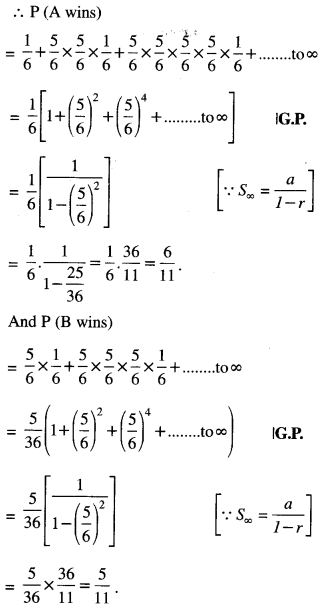Question 2.
If A and B are two independent events such that:
$$\mathbf{P}(\overline{\mathbf{A}} \cap \mathbf{B})=\frac{2}{\mathbf{1 5}}$$ and $$\mathbf{P}(\mathbf{A} \cap \overline{\mathbf{B}})=\frac{1}{\mathbf{6}}$$, then find P(A) and P(B). (C.B.S.E. 2015)
Solution:
Since A and B are independent events,
$$\overline{\mathrm{A}}$$ and B, A and $$\overline{\mathrm{B}}$$ are also independent events.
Now $$P(\bar{A} \cap B)=\frac{2}{15}$$ => $$\mathrm{P}(\overline{\mathrm{A}}) \mathrm{P}(\mathrm{B})=\frac{2}{15}$$
(1 – P(A)P(B) = $$\frac{2}{15}$$
P(B) – P(A))P(B) = $$\frac{2}{15}$$ ………… (1)
And P(A∩$$\overline{\mathrm{B}}$$)
P(A) $$\overline{\mathrm{B}}$$ = $$\frac{1}{6}$$
P(A) (1 — P(B)) = $$\frac{1}{6}$$
P(A)-P(A)P(B) = $$\frac{1}{6}$$ ………… (2)
Putting P(A) = x and P(B) = y in (1) and (2), we get
y – xy = $$\frac{2}{15}$$
and x – xy = $$\frac{1}{6}$$ ………..(2)
Subtracting (2) from (1) y = y – x = $$\frac{2}{15}$$ –$$\frac{1}{6}$$
⇒ y – x = $$\frac{-1}{30}$$
⇒ y = x – $$\frac{1}{30}$$ ………… (3)
Putting in (1)’, $$x-\frac{1}{30}-x\left(x-\frac{1}{30}\right)=\frac{2}{15}$$
⇒ $$x-\frac{1}{30}-x^{2}+\frac{x}{30}=\frac{2}{15}$$
⇒ 30x – 1 – 30x2 + x = 4
⇒ 30x2 – 31x + 5 = 0.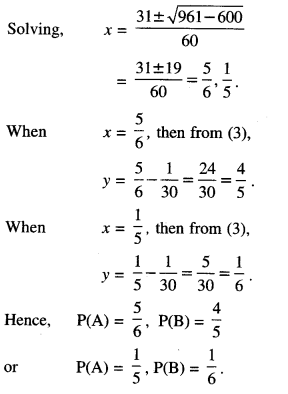If the girl tossed a coin .three times and exactly
1 tail shown, then: „ n
{HTH, HHT, THH} = 3
∴ P(A/E1) = $$\frac { 3 }{ 8 }$$

Let A be the event that the girl obtained ex-actly one tail. If the girl tossed a coin only once and exactly 1 tail.
∴ P(A/E2) = $$\frac { 1 }{ 2 }$$
By Bayes’ Theorem,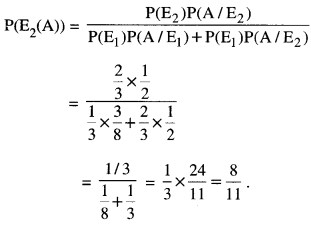Question 6.
A manufacturer has three machine operators A, B and C. The first operator A produces 1 % of defective items, whereas the other two operators B and C produce 5% and 7% defective items respectively. A is on the job for 50% of the time, B on the job 30% of the time and C on the job for 20% of the time. All the items are put into one stockpile and then one item is chosen at random from this and is found to be defective. What is the probability that it was produced by A?
(Delhi 2019)
Solution:
Let the events be as below :
E1 : Item produced by machine A.
E2 : Item produced by machine B.
E3 : Item produced by machine C. and D : Item drawn is defective.
We have to find P (E1/D).
Now P(E2) = $$\frac{50}{100}=\frac{1}{2}$$ ,
P(E2) = $$\frac{30}{100}=\frac{3}{10}$$
and P (E3) = $$\frac{20}{100}=\frac{1}{5}$$
Also P(D/E1) = $$\frac{1}{100}$$
P(D/E2) = $$\frac{5}{100}$$, P(D/E3) = $$\frac{7}{100}$$
By Bayes’ Theorem,
P(E1/D) =Question 7.
Bag I contain 3 white and 4 black balls, while Bag II contains 5 white and 3 black balls. One ball is transferred at random from Bag I to Bag II and then a ball is drawn at random from Bag II. The ball is drawn is found to be white. Find the probability that the transferred ball is also white.
(C.B.S.E. 2019 C)
Solution:
Let the events be as below:
E1 : 1 white ball is transferred from Bag I to Bag II
E2 : 1 black ball is transferred from Bag I to Bag II
and A: 1 white ball is drawn from Bag II.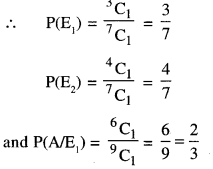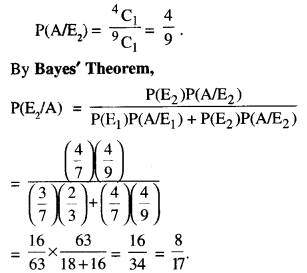Question 8.
There are three coins. One is a two-headed coin (having head on both the faces), another is a biased coin that comes up heads 75% of the time and the third is an unbiased coin. One of the three coins is chosen at random and tossed. If it shows head, what is the probability that it was the two-headed coin? (C.B.S.E. Sample Paper 2018-19)
Solution:
Let the events be:
E1 : coin is two headed
E2 : coin is biased and
E3 : coin is unbiased.
And, A : coin shows up head.
∴P(E1) = P(E2) = P(E3) = $$\frac{1}{3}$$
and P(A/E1) = 1, P(A/E2) = $$\frac{75}{100}=\frac{3}{4}$$
and P(A/E3) = $$\frac{1}{2}$$
By Bayes’ Theorem,
P(E1/A) =Hence, the required probability = $$\frac{4}{9}$$

Question 9.
Bag I contains 4 red and 2 green balls and Bag II contains 3 red and 5 green balls. One ball is transferred at random from Bag I to Bag II and then a ball is drawn at random from Bag II. The ball so drawn is found to be green in colour. Find the probability that the transferred ball is also green. (C.B.S.E. 2019 )
Solution:
Let the events be as below:
E1 : 1 red ball is transferred from Bag I to Bag II
E2 : 1 green ball is transferred from Bag I to Bag II
and A : 1 Green ball is drawn from Bag II.Question 10.
There are three coins. One is a coin having tails on both faces, another is a biased coin that comes up tails 70% of the time and the third is an unbiased coin. One of the coins is chosen at random and tossed, it shows tail. Find the probability that it was a coin with tail on both the faces. (Outside Delhi 2019)
Solution:
Let the events be as below :
E1 : Selected coin has tail on both faces
E2 : Selected coin is biased
E3 : Selected coin is unbiased and
A : Tail comes up.
Now P(E1 ) = P(E2 ) – P(E3 ) = $$\frac{1}{3}$$

P(A/E1) = 1,
P(A/E2) = $$\frac{70}{100}=\frac{7}{10}$$
P(A/E3) = $$\frac{1}{2}$$
By Bayes ’ Theorem :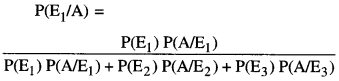Question 11.
Let a pair of dice be thrown and the random variable ‘X’ be the sum of the numbers that appear on the two dice. Find the mean (or expectation) of X. (N.C.E.R.T.)
Solution:
Clearly the sample space consists of 36 elementary events = {(xi, yi) ; xi, yi = 1, 2,…., 6}.
X, the random variable = Sum of the numbers on the two dice.
∴ ‘X’ takes values 2, 3,4, or 12.

Now P(X = 2) = P({1,1}) = $$\frac{1}{36}$$
P (X = 3) = P ({1,2}, {2,1}) =$$\frac{2}{36}$$
P (X = 4) = P {(1,3), (2,2), (3,1)} = $$\frac{3}{36}$$
P (X = 5) = P {(1,4),(2,3), (3,2),(4,1)} = $$\frac{4}{36}$$
P (X = 6) = P {(1,5), (2,4), (3,3), (4,2), (5,1)} = $$\frac{5}{36}$$
P (X = 7) = P {(1,6), (2,5), (3,4), (4,3), (5,2), (6,1)} = $$\frac{6}{36}$$
P (X = 8) = P {(2,6), (3,5), (4,4), (5, 3), (6,2)} = $$\frac{5}{36}$$
P (X = 9) = P {(3,6), (4,5), (5,4), (6,3)} = $$\frac{4}{36}$$
P (X = 10) = P {(4, 6), (5,5), (6,4)} = $$\frac{3}{36}$$
P (X = 11) = P {(5,6), (6,5)} = $$\frac{2}{36}$$
P (X = 12) = P {(6, 6} = $$\frac{1}{36}$$
Thus the probability distribution is:= $$\frac{1}{36}$$(2 + 6 + 12 + 20 + 30 + 42 + 40 + 36 + 30 + 22 + 12) = $$\frac{1}{36}$$ (252) = 7.
Hence, the required, mean = 7.

Question 12.
Find the mean and variance of the numbers obtained on a throw of an unbiased die.
(N.C.E.R.T.)
Solution:
Here sample space, S = {1, 2, 3, 4, 5, 6}.
Let ‘X’ denote the number obtained on the throw.
Thus ‘X’ takes values 1, 2, 3, 4, 5 or 6.
∴ P (1) = P (2) = = P(6) = $$\frac{1}{6}$$.
∴ Probability distribution is :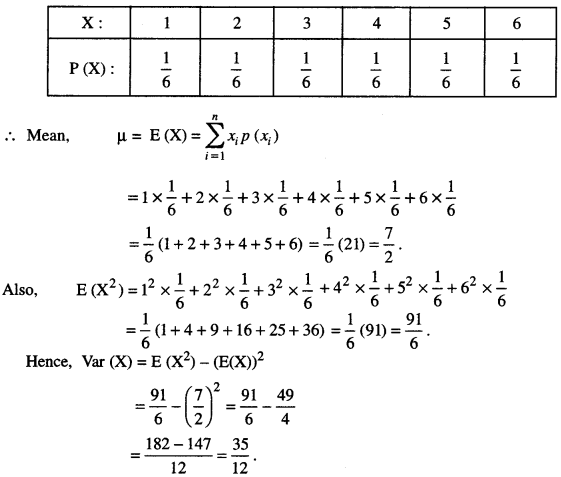Question 13.
Two cards are drawn simultaneously (or successively without replacement) from a well shuffled pack of 52 cards. Find the mean and variance of the number of kings. (C.B.S.E. Delhi 2019)
Solution:
Let X, number of kings = 0,1,2.
∴ P(X=0) = P (no king)
= $$\frac{48}{52} \times \frac{47}{51}=\frac{188}{221}$$
P(X = 1) = P (one king and one non-king)
= 2 x $$\frac{4}{52} \times \frac{48}{51}=\frac{32}{221}$$
P(X = 2) = P (two kings)
= $$\frac{4}{52} \times \frac{3}{51}=\frac{1}{221}$$
Hence, probability distribution is :